# Angular frequency facts for kids

Kids Encyclopedia Facts

In physics, angular frequency. ω. (also called the angular speed, radial frequency, and radian frequency) is a measure of rotation rate. A high rate of angular frequency means something is turning very fast. The angular frequency is the magnitude of the vector quantity angular velocity which is also known as the angular frequency vector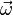$\vec{\omega}$.

It is useful in many areas of maths and science as it allows understanding of many properties of physical objects in our world. In SI units, angular frequency is measured in radians per second, with dimensions t−1 since radians are dimensionless.

## Theory

Angular freqency is often given in radians per second (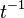$t^{-1}$) as it is easier to work with. In this way, the angular frequency is given by,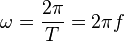$\omega = \frac{2\pi}{T} = 2 \pi f$

where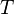$T$ is the time (period) of a single rotation(revolution) and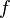$f$ is the frequency.

If a wheel turns by an angle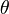$\theta$ in a time$t$ then the angular frequency at any moment is given by,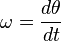$\omega = \frac{d\theta}{dt}$

If the rotation speed is constant, this becomes,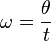$\omega = \frac{\theta}{t}$

and so it is similar to a speed.

For objects which are going in circles, the angular frequency may also be given as (in radians),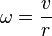$\omega = \frac{v}{r}$

where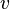$v$ is the speed and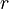$r$ is the distance from the middle of the circle.

## Examples

The angular frequency is important in determining whether an object can stay above the ground against gravity, or whether a spinning top can stay standing. It also is important in creating the frequency of mains electricity supplies and reducing the heat due to friction in engines.

### Satellites

Objects are pulled towards the earth because of gravity. To oppose this, a satellite must go fast enough it misses the ground. For the forces to equal each other,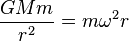$\frac{GMm}{r^2} = m \omega^2 r$

where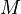$M$ is the mass of the larger body and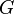$G$ is the Gravitational constant. To make a circle, the angular frequency must be equal to,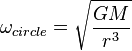$\omega_{circle} = \sqrt\frac{GM}{r^3}$

which leads to one of Johannes Kepler's Laws.

### Mains Supplies

Generators in power stations rotate at some frequency. The speed at which these turn gives us the frequency that our electricity supplies are at. These can be slowed by large brakes or big heavy wheels which stop the generators from turning too quickly.

## Units

### Examples of angular frequency measurements include

Name Symbol Definition
Radians per second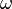$\omega$
Revolutions per minute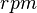$rpm$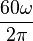$\frac{ 60 \omega }{2 \pi}$
Frequency per second$f$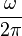$\frac{\omega}{2 \pi}$
Degrees per second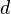$d$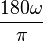$\frac{180 \omega}{\pi}$

## Images for kidsAngular frequency Facts for Kids. Kiddle Encyclopedia.